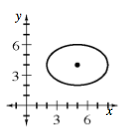### Home > A2C > Chapter 13 > Lesson 13.2.3 > Problem13-138

13-138.
1. Graph the following equations. Some of them will need to be changed into a more useful form. Homework Help ✎

1.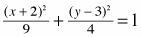2.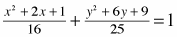3. 4(x2 − 10x + 25) + 9(y2 − 8y + 16) = 36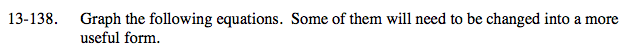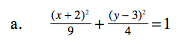Use the squared binomials to find the center and the denominators to find the edges of the graph.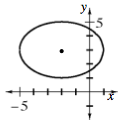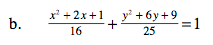Complete the squares for the numerators to find the center.

$\frac{(x+1)^2}{16}+\frac{(y+3)^2}{25}=1$

See part (a).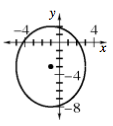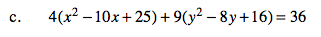Complete the squares.

4(x − 5)2 + 9(y − 4)2

Divide both sides by 36.

$\frac{(x-5)^2}{9}+\frac{(y-4)^2}{4}=1$

See part (a).